# Summation of divergent series

(diff) ← Older revision | Latest revision (diff) | Newer revision → (diff)

The construction of generalized sums of divergent series using summation methods. If, by means of a certain rule, to the series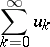(*)

a number has been attached, called the sum of the series, then one says that the series is summable to the sumby the summation method, or is-summable to the sum, and this fact is denoted by one of the symbols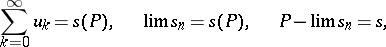where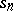are the partial sums of the series (*). In this case the numberis also called the-sum of the series. For example, for the series (*), the sequence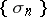of arithmetical averages of the firstpartial sums of the series,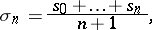can be examined. If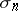has a limit when,then one says that the series (*) is summable to the sumby the summation method of arithmetical averages (cf. Arithmetical averages, summation method of), which is denoted by the symbol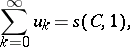orFor this definition of the sum of the series, every convergent series is summable to the sum to which it converges, and, moreover, there exist divergent series that are summable by this method. For example, the series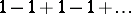is summable by the above method and its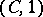-sum is equal to 1/2.

The definition of a summation method is usually subject to a series of requirements. For example, it is required that the method should sum a whole class of series; that it should not contradict convergence, i.e. that, being used for a convergent series, it should sum it to the same sum to which the series converges (see Regular summation methods); finally, that the summability of the series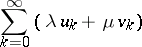to the sum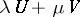should follow from the summability of the series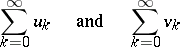by the given method to the sumsand, respectively (the linearity property). See also Divergent series.

How to Cite This Entry:
Summation of divergent series. Encyclopedia of Mathematics. URL: http://encyclopediaofmath.org/index.php?title=Summation_of_divergent_series&oldid=19002
This article was adapted from an original article by I.I. Volkov (originator), which appeared in Encyclopedia of Mathematics - ISBN 1402006098. See original article